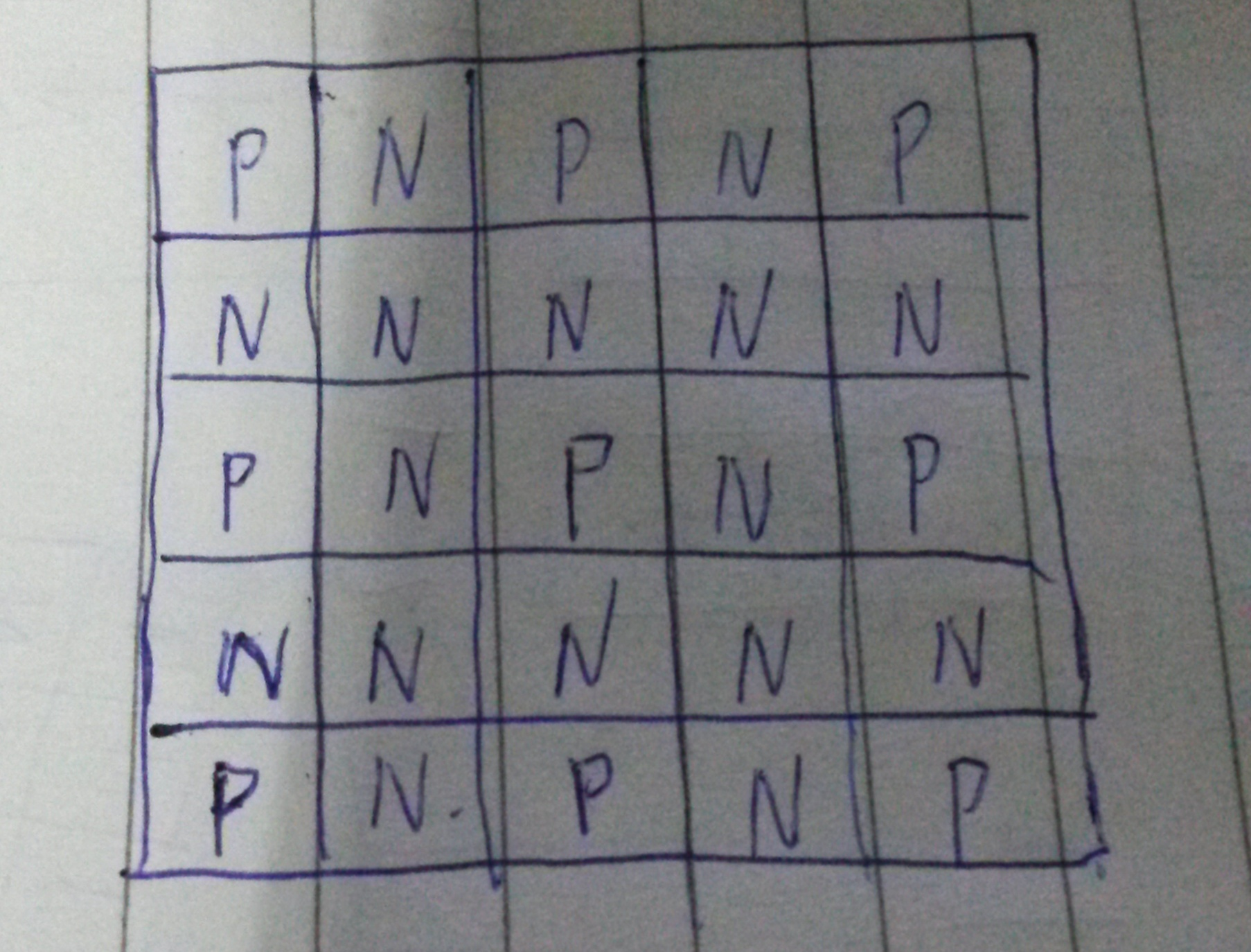1. 必胜状态后继节点一定有必败
2. 必败状态后继都是必胜

## 巴什博弈

x=n*(m+1)+s

### Brave game (HDU1846)

#include <bits/stdc++.h>
using namespace std;
int main(){
int t;
cin>>t;
while(t--){
int a,b;
cin>>a>>b;
int mod=a%(b+1);
if(mod>=1) cout<<"first"<<'\n';
else cout<<"second"<<'\n';
}
return 0;
}


### Public Sale (HDU2149)

#include <bits/stdc++.h>
using namespace std;
int main(){
int a,b;
while(cin>>b>>a){
int mod=b%(a+1);
if(a>=b){
for(int i=b;i<=a;i++){
if(i!=a) cout<<i<<' ';
else cout<<i<<'\n';
}
continue;
}
if(mod>=1) cout<<mod<<'\n';
else cout<<"none"<<'\n';
}
return 0;
}


1. 只能走到必胜点的是必败点
2. 可以走到必败点的是必胜点

**注意：**这里的必败必胜表示的是从这个点开始走的属性而不是走到这个点的属性

### kiki’s game (HDU2147)#include <bits/stdc++.h>
using namespace std;
int main(){
int n,m;
while(cin>>n>>m){
if(n==0 && m==0) break;
if(!(n&1) || !(m&1)) cout<<"Wonderful!"<<'\n';
else cout<<"What a pity!"<<'\n';
}
return 0;
}


## 斐波那契博弈

### 取石子游戏 (HDU2516)

#include <bits/stdc++.h>
using namespace std;
typedef long long ll;
int fbi;
int main(){
fbi=2;
fbi=3;
for(int i=3;i<=44;i++) fbi[i]=fbi[i-1]+fbi[i-2]; //注意用二分的话必须尺寸必须正好后面不能有0
int n;
while(cin>>n){
if(n==0) break;
int x=lower_bound(fbi+1,fbi+1+44,n)-fbi;
if(fbi[x]==n) cout<<"Second win"<<'\n';
else cout<<"First win"<<'\n';
}
return 0;
}


## 威佐夫博弈

$$\frac{(\sqrt{5}+1)}{2}*(y-x)=x$$

### 取石子游戏

#include <bits/stdc++.h>
using namespace std;
bool check(int a,int b){
int x=(b-a)*(sqrt(5)+1)/2;
if(x==a) return 1;
return 0;
}
int main()
{
int a,b;
while(cin>>a>>b){
if(a>b) swap(a,b);
if(check(a,b)) cout<<0<<'\n';
else cout<<1<<'\n';
}
return 0;
}


## Nim游戏

### Being a Good Boy in Spring Festival（HDU1850）

$$a1 \oplus a2 \oplus a3 \oplus a4 …=0(n>=i>=1)$$

#include <bits/stdc++.h>
using namespace std;
int a;
int main()
{
int n;
while(cin>>n){
if(n==0) break;
int nim=0;
for(int i=1;i<=n;i++){
cin>>a[i];
nim^=a[i];
}
if(nim==0){
puts("0");
continue;
}
int cnt=0;
for(int i=1;i<=n;i++){
int k=nim^a[i];
if(k<a[i]) cnt++;
}
cout<<cnt<<'\n';
}
return 0;
}


## SG函数

【实例】取石子问题

SG=0，f[]={1,3,4},

x=1 时，可以取走1 - f{1}个石子，剩余{0}个，所以 SG = mex{ SG }= mex{0} = 1;

x=2 时，可以取走2 - f{1}个石子，剩余{1}个，所以 SG = mex{ SG }= mex{1} = 0;

x=3 时，可以取走3 - f{1,3}个石子，剩余{2,0}个，所以 SG = mex{SG,SG} = mex{0,0} =1;

x=4 时，可以取走4- f{1,3,4}个石子，剩余{3,1,0}个，所以 SG = mex{SG,SG,SG} = mex{1,1,0} = 2;

x=5 时，可以取走5 - f{1,3,4}个石子，剩余{4,2,1}个，所以SG = mex{SG,SG,SG} =mex{2,0,1} = 3;

x 0 1 2 3 4 5 6 7 8…

SG[x] 0 1 0 1 2 3 2 0 1…

1、使用 数组f 将 可改变当前状态 的方式记录下来。

2、然后我们使用 另一个数组 将当前状态x 的后继状态标记。

3、最后模拟mex运算，也就是我们在标记值中 搜索 未被标记值 的最小值，将其赋值给SG(x)。

4、我们不断的重复 2 - 3 的步骤，就完成了 计算1~n 的函数值。

//f[N]:可改变当前状态的方式，N为方式的种类，f[N]要在getSG之前先预处理
//SG[]:0~n的SG函数值
//S[]:为x后继状态的集合
int f[N],SG[MAXN],S[MAXN];
void  getSG(int n){
int i,j;
memset(SG,0,sizeof(SG));
//因为SG始终等于0，所以i从1开始
for(i = 1; i <= n; i++){
//每一次都要将上一状态 的 后继集合 重置
memset(S,0,sizeof(S));
for(j = 1; f[j] <= i && j <= N; j++)
S[SG[i-f[j]]] = 1;  //将后继状态的SG函数值进行标记
for(j = 0;; j++) if(!S[j]){   //查询当前后继状态SG值中最小的非零值
SG[i] = j;
break;
}
}
}


#include <bits/stdc++.h>
using namespace std;
#define MAXN 1000 + 10
//int sg[MAXN];
//bool vis[MAXN];
//void init(int n){
//	sg=0;
//	sg=1;
//	for(int i=2;i<=n;i++){
//		memset(vis,0,sizeof vis);
//		for(int j=1;j<=i;j++){
//			vis[sg[i-j]]=1;
//			if(j!=i) vis[sg[i-j]^sg[j]]=1;
//		}
//		for(int j=0;;j++){
//			if(!vis[j]){
//				sg[i]=j;
//				break;
//			}
//		}
//	}
//}
int sg(int x){
if(x%4==0) return x-1;
if(x%4==3) return x+1;
return x;
}
int main(){
ios::sync_with_stdio(0); cin.tie(0); cout.tie(0);
//    init(100);
int t;
cin>>t;
while(t--){
int n;
cin>>n;
int c=0;
for(int i=1;i<=n;i++){
int tmp;
cin>>tmp;
c^=sg(tmp);
}
if(c==0) cout<<"Bob"<<'\n';
else cout<<"Alice"<<'\n';
}
return 0;
}

••